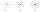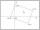# Analytic geometry - math word problems

Also known as coordinate geometry or Cartesian geometry.

#### Number of problems found: 126

• Find parametersFind parameters of the circle in the plane - coordinates of center and radius: ?
• SlopeCalculate the slope of a line that intersects points (-84,41) and (-76,-32).
• Ascend vs. descentWhich function is growing? a) y = 2-x b) y = 20 c) y = (x + 2). (-5) d) y = x-2
• V - slopeThe slope of the line whose equation is -3x -9 = 0 is
• Parametric equationPoint A [6; -2]. Point B = [-3; 1] Write the parametric equation of the line BA so that t belongs to the closed interval 0; 3
• SlopeWhat is the slope of a line with an inclination 6.06 rad?
• CircleFrom the equation of a circle: ? Calculate the coordinates of the center of the circle S[x0, y0] and radius of the circle r.
• ParabolaFind the equation of a parabola that contains the points at A[6; -5], B[14; 9], C[23; 6]. (use y = ax2+bx+c)
• AngleA straight line p given by the equation ?. Calculate the size of angle in degrees between line p and y-axis.
• Distance between 2 pointsFind the distance between the points (7, -9), (-1, -9)
• CircleWrite the equation of a circle that passes through the point [0,6] and touch the X-axis point [5,0]: ?
• Vertices of RTShow that the points P1 (5,0), P2 (2,1) & P3 (4,7) are the vertices of a right triangle.
• Square sideCalculate length of side square ABCD with vertex A[0, 0] if diagonal BD lies on line p: -4x -5 =0.
• Coordinates of square verticesThe ABCD square has the center S [−3, −2] and the vertex A [1, −3]. Find the coordinates of the other vertices of the square.
• Angle between vectorsFind the angle between the given vectors to the nearest tenth of a degree. u = (-22, 11) and v = (16, 20)
• Triangle IRTIn isosceles right triangle ABC with right angle at vertex C is coordinates: A (-1, 2); C (-5, -2) Calculate the length of segment AB.
• Vertex pointsGiven the following points of a triangle: P(-12,6), Q(4,0), R(-8,-6). Graph the triangle. Find the triangle area.
• Find the 13Find the equation of the circle inscribed in the rhombus ABCD where A[1, -2], B[8, -3] and C[9, 4].
• Find the 3Find the distance and midpoint between A(1,2) and B(5,5).
• Four sides of trapezoidTrapezoid is given by length of four sides: 40.5 42.5 52.8 35.0. Calculate its area.

Do you have an interesting mathematical word problem that you can't solve it? Submit a math problem, and we can try to solve it.

We will send a solution to your e-mail address. Solved examples are also published here. Please enter the e-mail correctly and check whether you don't have a full mailbox.

Please do not submit problems from current active competitions such as Mathematical Olympiad, correspondence seminars etc...

For Basic calculations in analytic geometry is a helpful line slope calculator. From coordinates of two points in the plane it calculate slope, normal and parametric line equation(s), slope, directional angle, direction vector, the length of segment, intersections the coordinate axes etc. See also more information on Wikipedia.